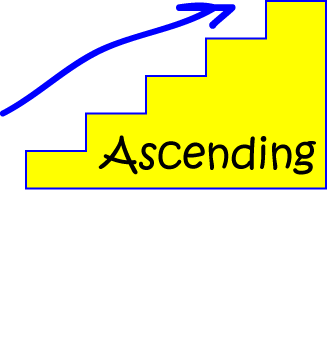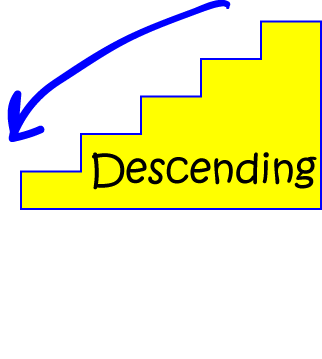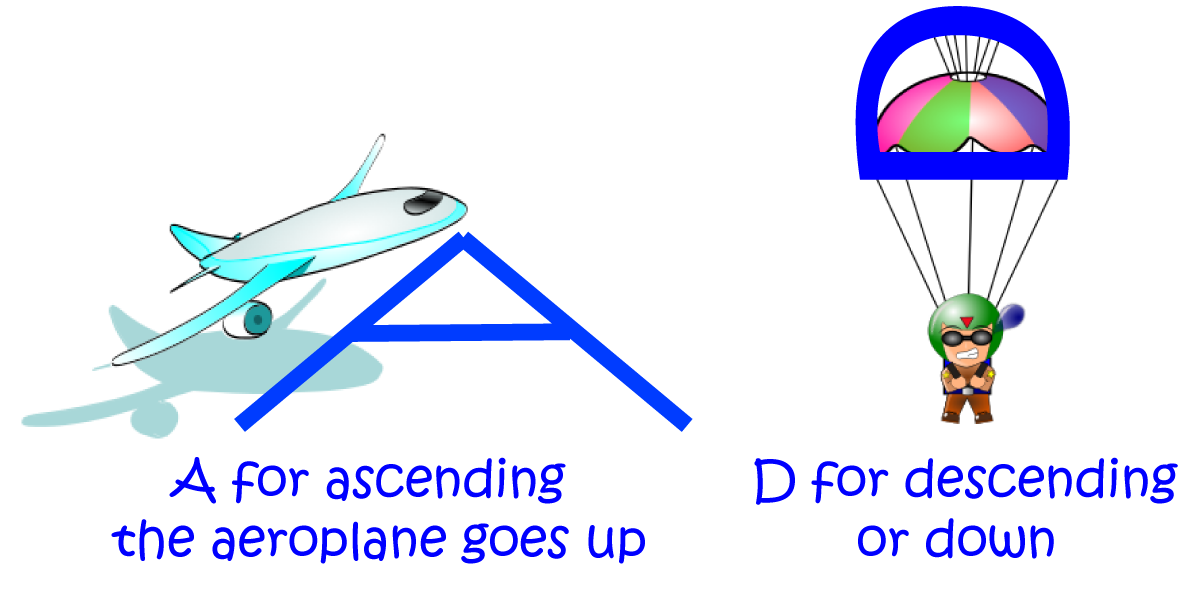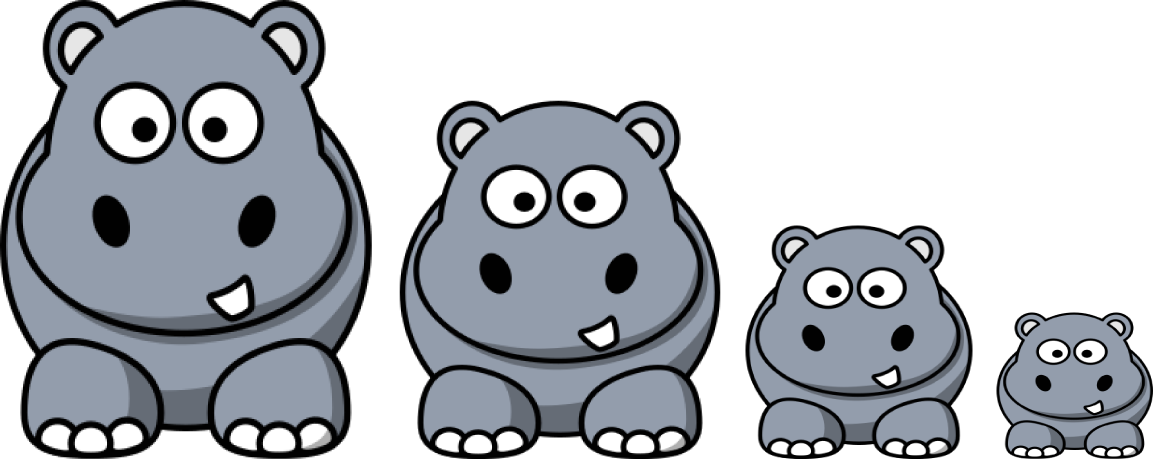# Ordering NumbersNo, not like that. Numbers have feelings too, you know!

## Ascending (Increasing) Order

To place numbers in ascending (or increasing) order, we put the smallest one first, then the next smallest one second, then the next next smallest one third, and so on. In other words, we write them from smallest to largest. Think of ascending a staircase, or the numbers increasing in size as we move from left to right.

### Example:

Place the numbers 3,9,2,23 and 11 in ascending order.

#### Solution:

Well, the smallest one in the list is 2, so it goes first. The second smallest one is 3, so it goes second. The third smallest is 9, so it goes third, the next smallest is 11, so it goes fourth, and the biggest is 23, so it goes last. Here's our list:
$2,3,9,11,23$.

### Another example:

Write the numbers 1,0,12,11,19 and 17 in increasing order.

#### Solution:

The smallest one is 0, so it goes first. Next comes 1. 11 is the next smallest number in the list, so it goes third. Then we have 12 and 17. 19 is the largest, so it goes last. Here's the list in increasing order:
$0,1,11,12,17,19$.

Increasing order and ascending order are just two different ways of saying the same thing.## Descending (Decreasing) Order

This is just the reverse of ascending or increasing order. To place numbers in descending (or decreasing) order, we put the largest one first, then the next largest one second, then the next next largest one third, and so on. In other words, we write them from largest to smallest. Think of descending a staircase, or the numbers decreasing in size as we move from left to right.

### Example:

Place the numbers 3,9,2,23 and 11 in descending order.

#### Solution:

Well, the largest one in the list is 23, so it goes first. The second largest one is 11, so it goes second. The third largest is 9, so it goes third, the next largest is 3, so it goes fourth, and the smallest is 2, so it goes last. Here's our list:
$23,11,9,3,2$.

### Another example:

Write the numbers 1,0,12,11,19 and 17 in decreasing order.

#### Solution:

The largest one is 19, so it goes first. Next comes 17. 12 is the next largest number in the list, so it goes third. Then we have 11 and 1. 0 is the smallest, so it goes last. Here's the list in decreasing order:
$19,17,12,11,1,0$.

Decreasing order and descending order are just two different ways of saying the same thing.## Remembering which is whichIt might help you to remember that the 'A' at the start of 'ascending' points upwards, and 'D' is for 'descending' and 'down'.

## Some more examples:

The elephants in the picture below are arranged in increasing (ascending) order of height, and probably of weight as well!The hippos in the picture below are arranged in decreasing (descending) order of height.## Some for you to try Measure your height and the heights of your friends. Write the heights you've found in decreasing order. You could also try to write your weights in increasing order. See if you can find the average weights of some of the animals in the zoo. For example, the average weight of a female giraffe is 830 kg, and wombats weigh somewhere between 20 and 35 kg. Arrange the animals in ascending order of weight. Annoy your friends by arranging the cards from an UNO deck in decreasing order and then asking them to have a game.

### Description

This mini book covers the core of Math for Foundation, Grade 1 and Grade 2 mathematics including

1. Numbers
3. Subtraction
4. Division
5. Algebra
6. Geometry
7. Data
8. Estimation
9. Probability/Chance
10. Measurement
11. Time
12. Money
13. and much more

This material is provided free of cost for Parent looking for some tricks for their Prekinder, Kinder, Prep, Year 1 and Year 2 children

### Learning Objectives

These lessons are for kids aged 4-8 with the core objective to expose their brains to concepts of addition, subtraction, division, algebra and much more.

Author: Subject Coach
You must be logged in as Student to ask a Question.